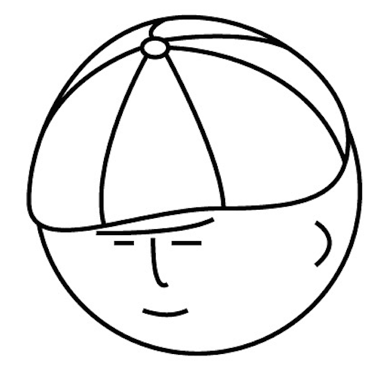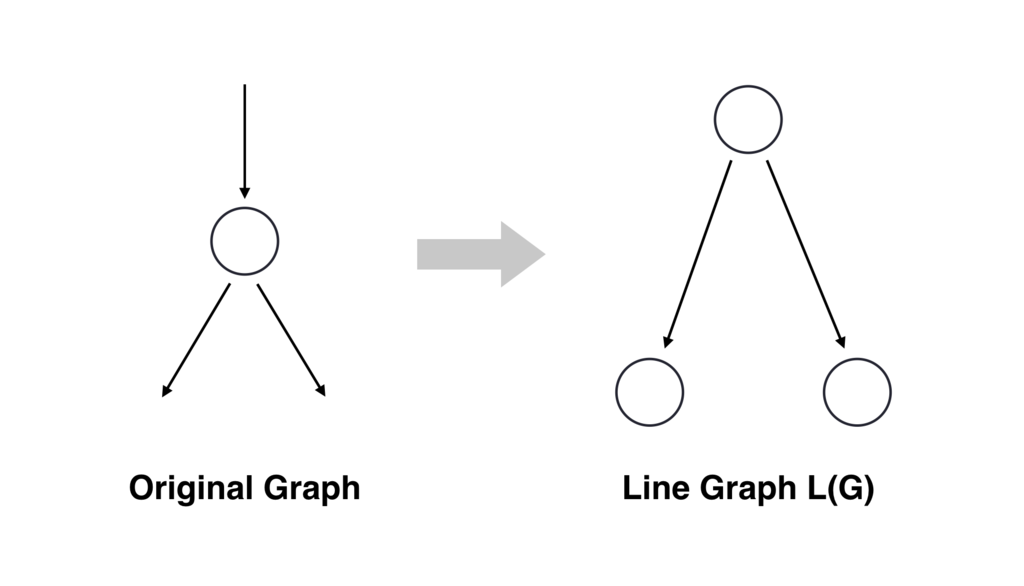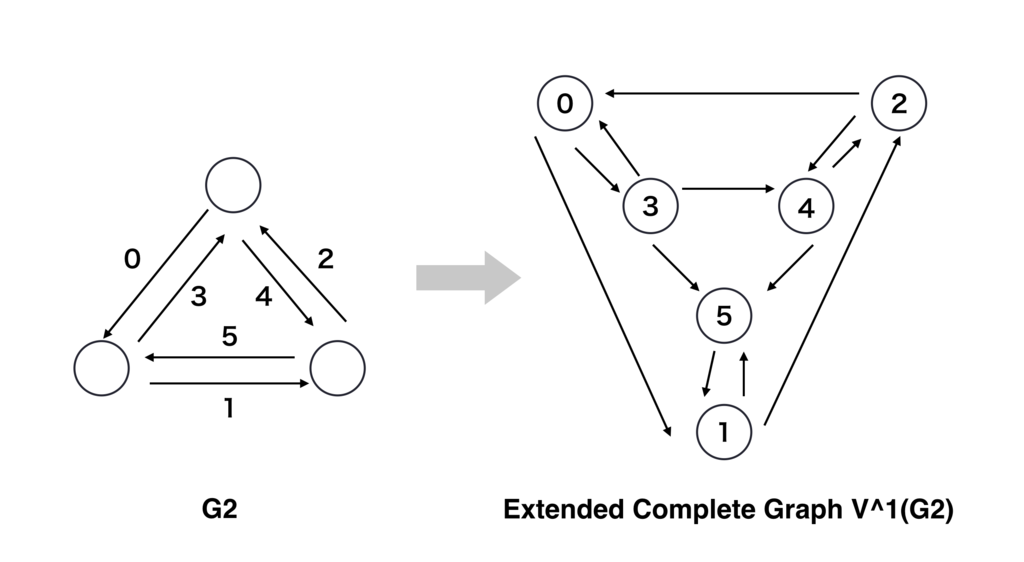# マツシタのお勉強メモ

#### マツシタのお勉強

##### welcome to my engineering blog
###### Hacker Rank# Connectivity and Damage Index

#### Connectivity

Connectivity express the reliability of the graph

• Node Connectivity Cv(G) : Minimum number of nodes to be removed to make a graph unconnected.
• Branch Connectivity Ce(G) : Minimum number of branches to be removed to make a graph unconnected.
• Node connectivity = d → d - 1 node failures OK
• Branch connectivity = d → d - 1 branch failures OK

#### Theorem1

Cv(G) ≦ Ce(G) ≦ δ(G) : δ = degree (the number of branches of one node)

#### Damage index

Damage index is an index of the increase of hops to connect in case of failure.

`α-Node(Branch) Damage Index Iα(G) (Jα(G))` is defined as blow

`Maximum diameter of a graph without α nodes(branches)` - `Diameter of the original graph G`

# Design Method of Extended Complete GraphExtended Complete Graph : Complete graph Gd → Line conversion, m times → Extended complete graph.

Lm(Gd) = L(L(…(L(G))…)) (m times)

Ex. 1Number of nodes Number of branched
Gd d+1 (d+1)d
L(Gd) (d+1)d (d+1)d2
L2(Gd) (d+1)d2 (d+1)d3

# Comparison

Complete graph Extended Complete Graph

Number of nodes `V` d + 1 (d + 1)dm
Number of branches `E` (d + 1)d (d+1)dm+1

Node connectivity Cv(G) d d
Branch connectivity Ce(G) d d

#### Throughput ratio in case of failure

k(G)/(Jα(G) + k(G)) → 0.5 for both Gd and Lm(Gd)